As the name suggests, our website is for students of any grade and all earnest Math learners

To prepare for Standardized Tests:

• Students who take various standardized tests such as SAT, GRE, GMAT, PCAT and other MBA and MCA tests in which Math is one important skill tested will find here relevant and useful math material on math subjects such as:

Algebra, Coordinate Geometry, Permutations and Combinations, Statistics, Calculus, Matrices, Vectors and Math subjects.

For Math Teachers, Colleges, Institutes and Universities.

• Math Teachers and Departments who wish to suggest their math students useful math websites

What math subjects and math lessons are/will be taken up in this website?

Algebra: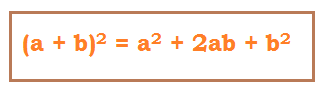Arithmetic: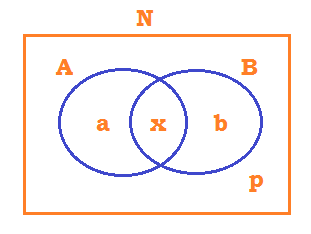Coordinate Geometry: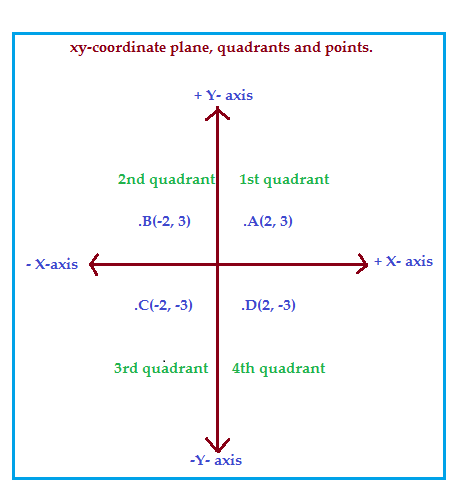Geometry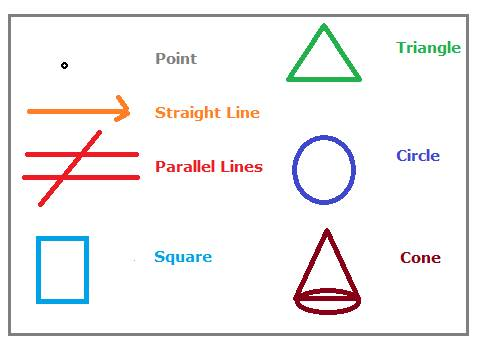Trigonometry: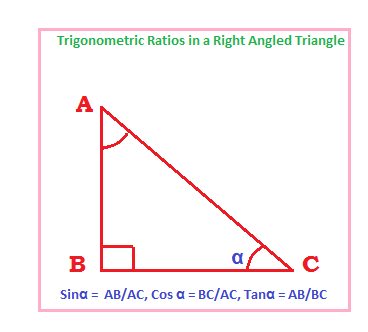Statistics: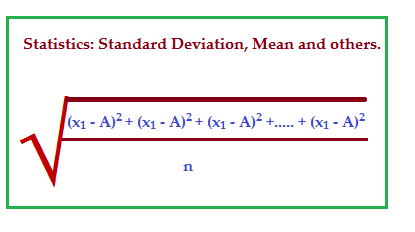Probability: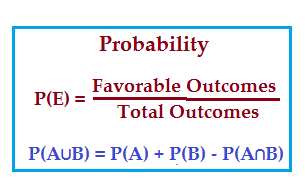Matrices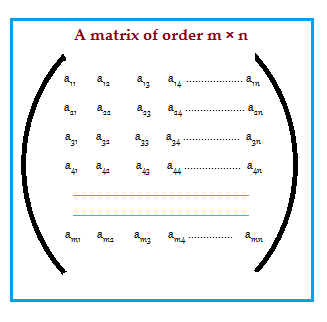How will math-for-all-grades explain the math subjects and math lessons? OR
What is the method of discussing the math subjects and lessons?

A coherent practical anecdote is presented to kindle interest and effortlessly lead the student into the actual topic.

• Links of the all of the math lessons under each major math topic are provided to take the student directly to the web page dealing with the lesson on clicking.
For example, under basic algebra > factorization, links of all major types of factorization are displayed, on clicking which, the student will be taken to the web page explaining the related lesson, say factoring trinomial perfect squares.

• A terse overview of all the math concepts in every math lesson is provided for two reasons

1. One, to give a bird’s eye view of the concepts to be discussed.
2. Another, more importantly, to serve a ready and quick recap of the salient concepts learnt in the specific math lesson.

Huge Number of Examples and Problems are solved

math-for-all-grades believes the key to mastery over math is to solve tens and scores of problems.

One Picture is worth a Thousand Words

• Wherever possible, appropriate images are included to illustrate the otherwise abstruse and uninteresting method of reading text – a necessary and indispensable mode of learning math.

there are buttons displayed under the Title: Math related. It contains:

o Math Glossary:

This gives a succinct definition of all important math terms and concepts in each math lesson.
The math glossary is exhibited topic wise. Alphabetical arrangement is also due for construction

o Math Formulas:

Math formulas will be arranged Subject-Wise. Math formulas on Algebra, Arithmetic, Trigonometry, Coordinate Geometry, Probability, Statistics, and Vectors will be presented for ready recap of the numerous important formulas in all math lessons in each math subject.

Students should practice and learn maths thoroughly as maths is so important. Mathematics underpins everything we do in life. Even bitcoin, the popular cryptocurrency, is based on mathematics. Cryptocurrency can provide its owner with numerous advantages. Trading with bitcoin might help investors generate more money if they use a reliable crypto trading app. Consider going to a website that has already completed the trading app test and found out the finest cryptocurrency trading apps available right now.